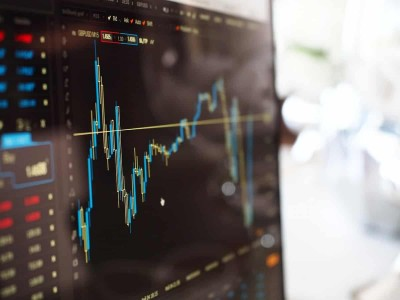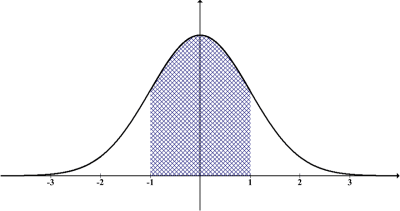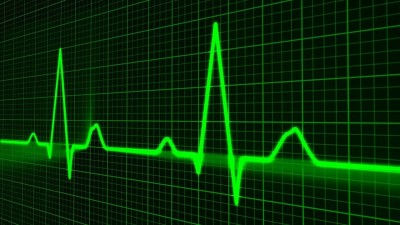# Where Is Standard Deviation Used? (8 Real Life Examples)

After you learn how to calculate standard deviation and what it can tell you, the next logical step is to find out when this concept becomes useful.

So, where is standard deviation used in real life?  Standard deviation tells us the variability of a data set in several applications, including: academia, business, finance, forecasting, manufacturing, medicine, polling, and population traits. It can also be used for tools like coefficient of variation, hypothesis testing, and confidence intervals.

Of course, standard deviation is a tool for analysis, just like median, expected value, and other statistical measures.  We need to know how to use it properly and interpret it before we apply the concept.

In this article, we’ll talk about standard deviation and where it is used in real life.  We’ll give some examples for some of these applications so that you can see some hard numbers.

Let’s get started.

Having math trouble?

Looking for a tutor?

## Where Is Standard Deviation Used? (8 Real Life Examples)

Standard deviation is used to measure the variability of values in a data set.  It has a wide range of applications in academia, business, and science, including:

• Academic Studies (coefficient of variation, hypothesis testing, confidence intervals)
• Business (variability of delivery times, inventory, etc.)
• Finance (such as variability of returns in different asset classes)
• Forecast Accuracy (such as weather)
• Manufacturing (quality control, precision machining of parts to ensure proper size)
• Medicine (effectiveness of drugs in pharmaceutical trials)
• Polling (margin of error for opinion polls)
• Population Traits (height, weight, IQ, etc.)

Let’s start off with applications of standard deviation in academic studies.

### Standard Deviation In Academic Studies

Standard deviation is used for academic studies in various fields such as:

• Applied Mathematics & Statistics
• Biology
• Chemistry
• Psychology

On its own, standard deviation can give us an idea of how “spread out” a data set is about the mean.  This is because standard deviation is a measure of dispersion.

Two data sets could have the same mean and different standard deviations if one data set has values that are more spread out. (You can learn more about the difference between mean and standard deviation in my article here).

Standard deviation gives us an idea of how much variability to expect:

• High standard deviation means the average data point is far away from the mean.  That is, the data is fairly spread out from the mean.
• Low standard deviation means the average data point is relatively close to the mean.  That is, the data is not spread out too far from the mean.
• Zero standard deviation means that every data point is the same (there is no variability).  This case is rare, but it is possible.

We can also use standard deviation together with mean to look at additional parameters and methods, such as coefficient of variation, hypothesis testing, and confidence intervals.

#### Coefficient Of Variation

The coefficient of variation (CV) of a data set is defined as:

• CV = S / M

where S is the standard deviation of the data set and M is its mean (average).

The coefficient of variation can give us an idea of how the standard deviation compares to the mean:

• A CV of less than 1 means that the standard deviation is low.  It also tells us that the standard deviation is less than the mean.
• A CV of more than 1 means that the standard deviation is high.  It also tells us that the standard deviation is greater than the mean.

For example, let’s say we have two data sets:

• Data Set A has a mean of 10 and a standard deviation of 2.
• Data Set B has a mean of 1 and a standard deviation of 2.

Although the standard deviation is the same for both data sets, the coefficient of variation will differ quite a bit:

• CV for Data Set A: S / M = 2 / 10 = 0.2
• CV for Data Set B: S / M = 2 / 1 = 2

So, the standard deviation of 2 is considered low for data set A, but it is considered high for data set B.

It is important to note that a mean close to zero will skew the coefficient of variation to a high value.  Even worse, a mean of zero implies an undefined coefficient of variation (due to a zero denominator).

The sample size will also affect sample standard deviation, which can influence the coefficient of variation.

#### Hypothesis Testing

Another important use of standard deviation in academia (and in other fields) is hypothesis testing.  Basically, there are 4 steps:

• 1. Formulate the null hypothesis, H0.
• 2. Formulate the alternative hypothesis, Ha.
• 3. Calculate a test statistic.
• 4. Determine whether you reject or fail to reject the null hypothesis H0 based on the value of the test statistic.

For a population that is normally distributed, we will often calculate the test statistic Z = (X – M) / S.  This means taking the value we wish to test, subtracting the population mean, and dividing by the population standard deviation.

The result gives us a standard normal distribution (which can have negative values), with a mean of 0 and a standard deviation of 1.  We can then use a standard normal table of values to find the percentile (percent chance) that the given value would occur.

#### Confidence Intervals

We can come up with a range of values (a confidence interval) at 90%, 95%, or higher confidence levels.  This gives us a reasonable degree of certainty that a value falls within a certain range.

For example, a 95% confidence interval of (50, 60) for the temperature (in degrees Fahrenheit) means that the true temperature will fall within the range (50, 60) 95% of the time.

### Standard Deviation In Finance

Within the field of finance, we can use standard deviation to determine the volatility (or variability) of returns from investments in various asset classes, including:

• Stocks (including both dividends and capital appreciation)
• Bonds (including corporate, federal, and municipal)
• Commodities (such as gold and silver)
• Real Estate (including investment properties and REITs)When you analyze rates of return in real estate (or any other investment), consider the variability of returns in addition to the “average” return.

The mean rate of return tells us what to expect from an investment in an average year.  However, we can get more information when we look at the standard deviation of those returns over the long run.

For example, let’s say we have the chance to invest in two different stocks (for the sake of argument, let’s say we want to choose only one to invest in, just to keep things simple).

The stocks have the following historical performance over 10 years:

• Stock A: mean annual return = 7%, standard deviation of annual returns = 7%
• Stock B: mean annual return = 7%, standard deviation of annual returns = 2%

Both stocks have the same average rate of return, but the volatility is much higher for Stock A.  The stock you choose to invest in will depend on your tolerance for volatility of returns (risk).

If you are fine with the possibility of negative returns in exchange for a good chance at double digit returns, then you would choose to invest in Stock A.

If you would like to preserve your capital and avoid negative returns, then you would choose to invest in Stock B.

Note: if you wish to get an average return of 7% with a standard deviation between 2% and 7%, you could buy some shares in both Stock A and Stock B to balance your portfolio.The standard deviation of stock market returns is just as important to consider as mean of returns.

Having math trouble?

Looking for a tutor?

### Standard Deviation In Forecast Accuracy

When making a forecast (such as weather predictions) or a projection (such as annual company revenue), there is both an expected value (the projection) and variability (the error or uncertainty).

For example, if we look at 10 years of historical weather data for a particular day in a particular city, we might find a mean high temperature of 70 degrees Fahrenheit and a standard deviation of 2 degrees Fahrenheit.

Our best guess (without any other information) is that the high temperature will be 70 degrees Fahrenheit on that day this year.  However, we can also make some guesses involving ranges (based on the assumption of a normal distribution):

• The high temperature will be in the range (68, 72) in 68% of the years.
• The high temperature will be in the range (66, 74) in 95% of the years.
• The high temperature will be in the range (64, 76) in 99.7% of the years.

Of course, if we do have additional information (such as cold fronts and storms moving through the area), then we can revise our projections with this new data.

### Standard Deviation In Manufacturing

In manufacturing, there is a certain tolerance for defects, but the closer we can get to the specified dimensions, the better.  For projects that require low variability (small parts with precise specifications), it will be important to keep standard deviation low.

Of course, the tradeoff for quality is either time or cost.  A machine (or machinist) that can do more precise work will cost more money or will take more time (or both).

For example, let’s say we have a manufacturing process with a mean width of 0.5 inches and a standard deviation of 0.01 inches.  Let’s also assume the size of the manufactured items follows a normal distribution.

Then we would expect:

• 68% of the items to fall in the range (0.49, 0.51) inches, or within 0.01 inches of the 0.5 inch specification.
• 95% of the items to fall in the range (0.48, 0.52) inches, or within 0.02 inches of the 0.5 inch specification.
• 99.7% of the items to fall in the range (0.47, 0.53) inches, or within 0.03 inches of the 0.5 inch specification.

If a customer specifies that 95% of the items must be within 0.02 inches of the specified 0.5 inch size, then we will be able to fulfill the order.

If a customer specified that 99% of the items must be within 0.03 inches of the specified 0.5 inch size, then we will also be able to fulfill that order.

However, if a customer specifies that 90% of the items must be within 0.01 inches of the specified 0.5 inch size, then we will not be able to fulfill the order with our current capabilities.A normal distribution is symmetric about the mean (here, the standard normal has a mean of zero and a standard deviation of 1).

### Standard Deviation In Medicine

Standard deviation can give us more information about a group of people in a medical trial than the sample mean alone.  Standard deviation tells us how dispersed the values in a data set are.

For example, two groups could have the same mean cholesterol level, but have vastly different standard deviations.  This could be due to lifestyle factors of participants in a trial:

• Group A might be made up of people in reasonably good shape – they are not elite athletes, but they are not couch potatoes either.  Their mean total cholesterol might be 180 mg/dL, and the standard deviation might be 10 mg/dL.
• Group B might have a more diverse group of people: some of whom take great care of themselves, and others who do not.  Although their mean total cholesterol might also be 180 mg/dL, the standard deviation might be 40 mg/dL.

Due to the low standard deviation, the people in group A are in relatively the same shape (as far as cholesterol goes).  The high standard deviation of group B tells us that the healthy and unhealthy people in that group have a much bigger difference in their cholesterol levels.Standard deviation of cholesterol levels (or other measures) can tell us about the variability of the health of a group of people.

### Standard Deviation In Polling

When taking opinion polls, we are interested in what the final percentages say.  However, we are also interested in how certain we are that we can draw a conclusion from those percentages.

For example, let’s say a poll suggests that 52% of voters support a new law, with a margin of error of 4%.  This means that the percentage of voters supporting the new law is really in the interval

• (52% – 4%, 52% + 4%)
• (48%, 56%)

Although it is more likely than not that a majority (>50%) of voters support the new law, it is still possible that a majority are in opposition.

Of course, all of this assumes that the sample of people polled is representative of the population at large.  If you poll exclusively in one state, then that will not be representative of the entire United States.The margin of error in polling depends on the standard deviation (which depends on sample size).

### Standard Deviation In Population Traits

We might be interested in both the mean and standard deviation for certain population traits, such as:

• Height
• Weight
• IQ

When we calculate both the mean and standard deviation, we can figure out what percentage of people are within a certain range of values.

For example, if the mean IQ is M = 100 and the standard deviation is S = 10, then we know that:

• 68% of people are within one standard deviation of the mean: (M – S, M + S) = (90, 110).
• 95% of people are within one standard deviation of the mean: (M – 2S, M + 2S) =  (80, 120).
• 99.7% of people are within one standard deviation of the mean: (M – 3S, M + 3S) = (70, 130).

We can also calculate other percentages.  For example, 34% of people have an IQ between 100 and 110.

## Conclusion

Now you know where standard deviation is used in real life and how it is used in these applications.

You can learn about the units for standard deviation here.